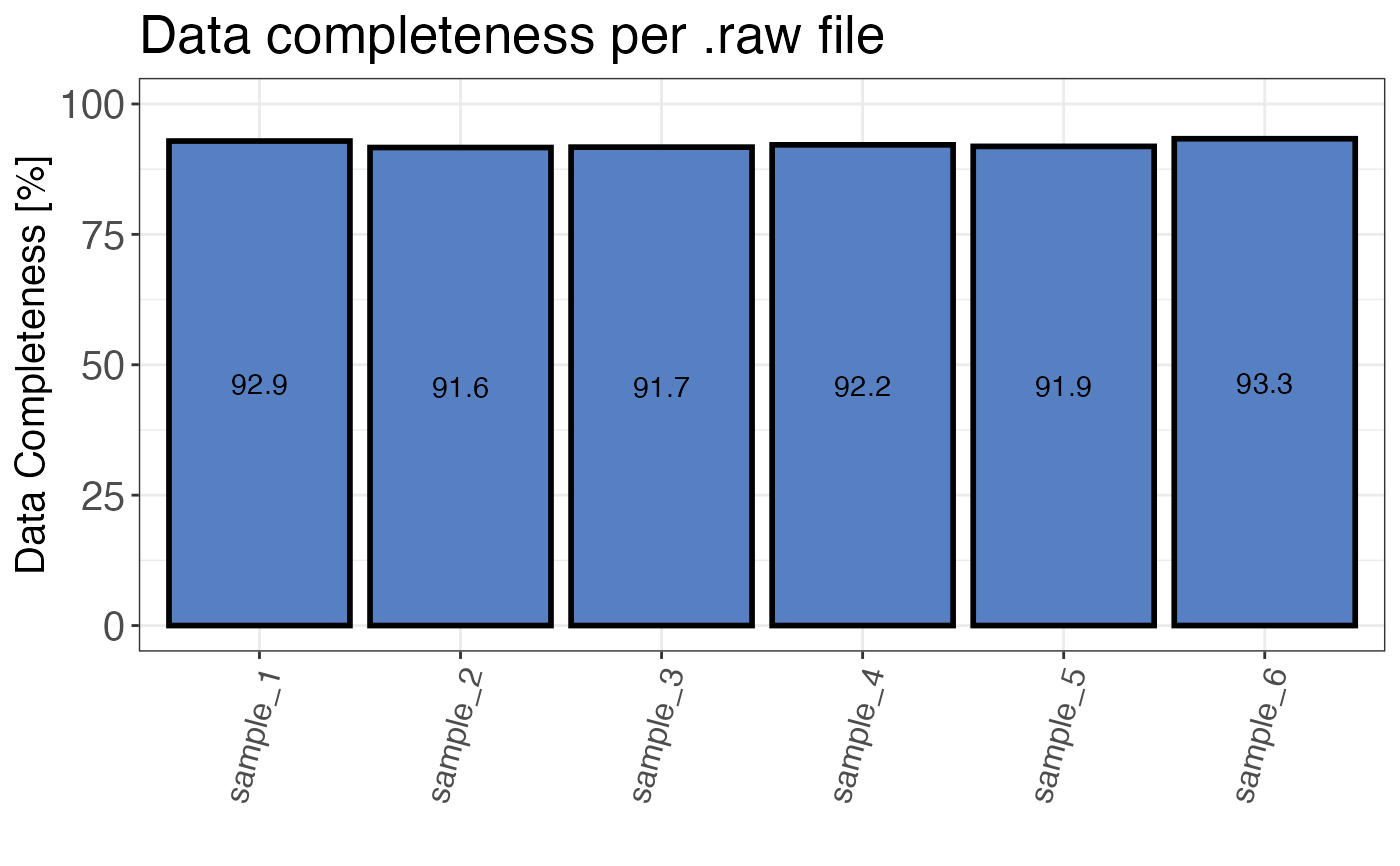Calculates the percentage of data completeness. That means, what percentage of all detected precursors is present in each sample.

qc_data_completeness(
data,
sample,
grouping,
intensity,
digestion = NULL,
plot = TRUE,
interactive = FALSE
)

## Arguments

data a data frame containing at least the input variables. a character column in the data data frame that contains the sample names. a character column in the data data frame that contains either precursor or peptide identifiers. a numeric column in the data data frame that contains any intensity intensity values that missingness should be determined for. optional, a character column in the data data frame that indicates the mode of digestion (limited proteolysis or tryptic digest). Alternatively, any other variable by which the data should be split can be provided. a logical value that indicates whether the result should be plotted. a logical value that specifies whether the plot should be interactive (default is FALSE).

## Value

A bar plot that displays the percentage of data completeness over all samples. If plot = FALSE a data frame is returned. If interactive = TRUE, the plot is interactive.

## Examples

set.seed(123) # Makes example reproducible

# Create example data
data <- create_synthetic_data(
n_proteins = 100,
frac_change = 0.05,
n_replicates = 3,
n_conditions = 2,
method = "effect_random"
)

# Determine data completeness
qc_data_completeness(
data = data,
sample = sample,
grouping = peptide,
intensity = peptide_intensity_missing,
plot = FALSE
)
#> # A tibble: 6 × 2
#>   sample   completeness
#>   <chr>           <dbl>
#> 1 sample_1         92.9
#> 2 sample_2         91.6
#> 3 sample_3         91.7
#> 4 sample_4         92.2
#> 5 sample_5         91.9
#> 6 sample_6         93.3
# Plot data completeness
qc_data_completeness(
data = data,
sample = sample,
grouping = peptide,
intensity = peptide_intensity_missing,
plot = TRUE
)